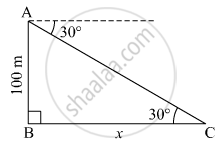# From the Top of the Light House, an Observer Looks at a Ship and Finds the Angle of Depression to Be 30°. If the Height of the Light-house is 100 Meters, Then - Geometry

#### Question

From the top of the light house, an observer looks at a ship and finds the angle of depression to be 30°. If the height of the light-house is 100 meters, then find how far the ship is from the light-house.

#### Solution

Let AB be the lighthouse and C be the position of the ship from the lighthouse.
Suppose the distance of the ship from the lighthouse be x m.Here, AB = 100 m and ∠ACB = 30º.
In right ∆ABC,
$\tan30^\circ = \frac{AB}{BC}$
$\Rightarrow \frac{1}{\sqrt{3}} = \frac{100}{x}$
$\Rightarrow x = 100\sqrt{3} m$
Thus, the ship is $100\sqrt{3}$ m away from the lighthouse.

Is there an error in this question or solution?

#### Video TutorialsVIEW ALL 

From the Top of the Light House, an Observer Looks at a Ship and Finds the Angle of Depression to Be 30°. If the Height of the Light-house is 100 Meters, Then Concept: Heights and Distances.